# January 2010

Here is this month’s selection of Traders’ Tips, contributed by various developers of technical analysis software to help readers more easily implement some of the strategies presented in this and other issues.

Other code appearing in articles in this issue is posted in the Subscriber Area of our website at http://technical.traders.com/sub/sublogin.asp. Login requires your last name and subscription number (from mailing label). Once logged in, scroll down to beneath the “Optimized trading systems” area until you see “Code from articles.” From there, code can be copied and pasted into the appropriate technical analysis program so that no retyping of code is required for subscribers.

You can copy these formulas and programs for easy use in your spreadsheet or analysis software. Simply “select” the desired text by highlighting as you would in any word processing program, then use your standard key command for copy or choose “copy” from the browser menu. The copied text can then be “pasted” into any open spreadsheet or other software by selecting an insertion point and executing a paste command. By toggling back and forth between an application window and the open web page, data can be transferred with ease.

This month’s tips include formulas and programs for:TRADESTATION: VORTEX INDICATOR

Etienne Botes and Douglas Siepman’s article in this issue, “The Vortex Indicator,” describes an indicator intended to identify price trends. The authors include some trading rules. Code for the indicator and for a strategy based on the trading rules is shown here.

Both the indicator and strategy use a parameter named length to define the number of price bars over which to perform certain calculations. The strategy also uses a trailing stop factor, which is the number of average true ranges at which to trail an exit stop order.

To download the adapted EasyLanguage code, go to the TradeStation and EasyLanguage Support Forum (https://www.tradestation.com/Discussions/forum.aspx?Forum_ID=213). Search for the file “Vortex.eld.”

A sample chart is shown in Figure 1.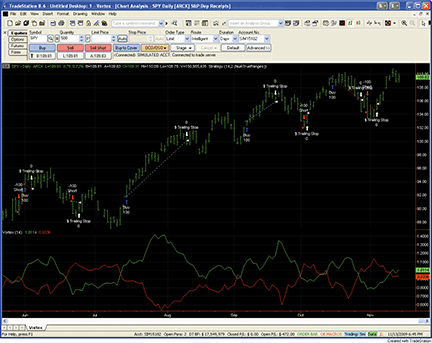Figure 1: TRADESTATION, VORTEX INDICATOR. Here is an example of the Vortex Indicator and strategy applied to a daily chart of SPY.

```Indicator:  Vortex
inputs:
Length( 14 ) ;

variables:
VMPlus( 0 ),
VMMinus( 0 ),
VMPlusSum( 0 ),
VMMinusSum( 0 ),
TR( 0 ),
TRSum( 0 ),
VIPlusSumRge( 0 ),
VIMinusSumRge( 0 ) ;

VMPlus = AbsValue( High - Low ) ;
VMMinus = AbsValue( Low - High ) ;
VMPlusSum = Summation( VMPlus, Length ) ;
VMMinusSum = Summation( VMMinus, Length ) ;
TR = TrueRange ;
TRSum = Summation( TR, Length ) ;

if TRSum <> 0  then
begin
VIPlusSumRge = VMPlusSum / TRSum ;
VIMinusSumRge = VMMinusSum / TRSum ;
end ;

Plot1( VIPlusSumRge, "VI+Sum/Rge", Green ) ;
Plot2( VIMinusSumRge, "VI-Sum/Rge", Red ) ;

Strategy:  VortexStrat
inputs:
Length( 14 ),
StopFactor( 2 ) ; { number of true ranges }

variables:
VMPlus( 0 ),
VMMinus( 0 ),
VMPlusSum( 0 ),
VMMinusSum( 0 ),
TR( 0 ),
TRSum( 0 ),
VIPlusSumRge( 0 ),
VIMinusSumRge( 0 ),
ShortSignal( false ),
StopPrice( 0 ) ;

VMPlus = AbsValue( High - Low ) ;
VMMinus = AbsValue( Low - High ) ;
VMPlusSum = Summation( VMPlus, Length ) ;
VMMinusSum = Summation( VMMinus, Length ) ;
TR = TrueRange ;
TRSum = Summation( TR, Length ) ;

if TRSum <> 0  then
begin
VIPlusSumRge = VMPlusSum / TRSum ;
VIMinusSumRge = VMMinusSum / TRSum ;
end ;

if VIPlusSumRge crosses over VIMinusSumRge then
begin
BuySignal = true ;
ShortSignal = false ;
StopPrice = High ;
end
else if VIPlusSumRge crosses under VIMinusSumRge then
begin
BuySignal = false ;
ShortSignal = true ;
StopPrice = Low ;
end ;

MarketPosition <> 1 then
Buy next bar StopPrice stop ;

if ShortSignal and TotalTrades = SignalTradeNum and
MarketPosition <> -1 then
SellShort next bar at StopPrice stop ;

SetStopShare ;
SetDollarTrailing( TR * StopFactor ) ;
```

This article is for informational purposes. No type of trading or investment recommendation, advice, or strategy is being made, given, or in any manner provided by TradeStation Securities or its affiliates.

—Mark Mills
A subsidiary of TradeStation Group, Inc.

BACK TO LISTeSIGNAL: VORTEX INDICATOR

For this month’s Traders’ Tip, we’ve provided the formula “Vortex.efs” based on the code in Etienne Botes and Douglas Siepman’s article in this issue titled “The Vortex Indicator.”

The study contains two formula parameters: length of vortex, and length of true range. These may be configured through the Edit Studies window (Advanced Chart menu -> Edit Studies).

A sample chart is shown in Figure 2.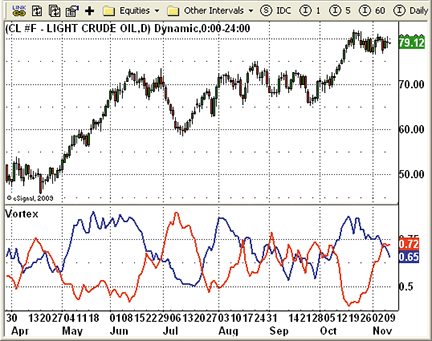Figure 2: eSIGNAL, VORTEX INDICATOR. Here is a demonstration of the Vortex Indicator with a vortex period length of 14 and a true range period length of 21 on light crude oil futures.

```/*********************************
Provided By:
eSignal (Copyright c eSignal), a division of Interactive Data
Formula Script (EFS) is for educational purposes only and may be
modified and saved under a new file name.  eSignal is not responsible
for the functionality once modified.  eSignal reserves the right
to modify and overwrite this EFS file with each new release.

Description:
The Vortex Indicator
Version:            1.01  12/29/2009

Formula Parameters:                     Default:
Length of Vortex                    14
Notes:
The related article is copyrighted material. If you are not a subscriber
of Stocks & Commodities, please visit www.traders.com.

**********************************/
var fpArray = new Array();
var bInit = false;
var bVersion = null;

function preMain() {
setPriceStudy(false);
setShowCursorLabel(true);
setShowTitleParameters(false);
setStudyTitle("Vortex");
setCursorLabelName("+VI", 0);
setDefaultBarFgColor(Color.blue, 0);
setPlotType(PLOTTYPE_LINE, 0);
setDefaultBarThickness(2, 0);
setCursorLabelName("-VI", 1);
setDefaultBarFgColor(Color.red, 1);
setPlotType(PLOTTYPE_LINE, 1);
setDefaultBarThickness(2, 1);
var x=0;
fpArray[x] = new FunctionParameter("LengthVortex", FunctionParameter.NUMBER);
with(fpArray[x++]){
setName("Length of Vortex");
setLowerLimit(1);
setDefault(14);
}
}

var xVortexPlus = null;
var xVortexMinus = null;

function main(LengthVortex) {
var nBarState = getBarState();
var nVortexP = 0;
var nVortexM = 0;
if (bVersion == null) bVersion = verify();
if (bVersion == false) return;
if (nBarState == BARSTATE_ALLBARS) {
if (LengthVortex == null) LengthVortex = 14;
}
if (!bInit) {
xVortexPlus = efsInternal("Calc_Vortex", LengthVortex);
xVortexMinus = getSeries(xVortexPlus, 1);
bInit = true;
}
nVortexP = xVortexPlus.getValue(0);
nVortexM = xVortexMinus.getValue(0);
if (nVortexP == null || nVortexM == null) return;
return new Array(nVortexP, nVortexM);
}

var bSecondInit = false;
var xVMm = null;
var xVMp = null;
var xVM_TrueRange = null;

function Calc_Vortex(LengthVortex) {
var nVortexP = 0;
var nVortexM = 0;
var nVMpSum = 0;
var nVMmSum = 0;
var nTRSum = 0;
var i = 0;
if (bSecondInit == false) {
xVMp = efsInternal("Calc_VM_TR");
xVMm = getSeries(xVMp, 1);
xVM_TrueRange = getSeries(xVMp, 2);
bSecondInit = true;
}
if (xVMp.getValue(-LengthVortex) == null || xVM_TrueRange.getValue(-LengthVortex) == null) return;
for (i = LengthVortex; i >= 0; i--) {
nVMpSum += xVMp.getValue(-i);
nVMmSum += xVMm.getValue(-i);
nTRSum += xVM_TrueRange.getValue(-i);
}
if (nTRSum != 0) {
nVortexP = nVMpSum / nTRSum;
nVortexM = nVMmSum / nTRSum;
}
return new Array(nVortexP, nVortexM);
}

var bThirdInit = false;
var xHigh = null;
var xLow = null;
var xClose = null;

function Calc_VM_TR() {
var nVMp = 0;
var nVMm = 0;
var nH1 = 0;
var nH = 0;
var nL1 = 0;
var nL = 0;
var nC1 = 0;
var nTR = 0;
if (bThirdInit == false) {
xHigh = high();
xLow = low();
xClose = close();
bThirdInit = true;
}
nH1 = xHigh.getValue(-1);
nH = xHigh.getValue(0);
nL1 = xLow.getValue(-1);
nL = xLow.getValue(0);
nC1 = xClose.getValue(-1);
if (nH1 == null) return;
nVMp = Math.abs(nH - nL1);
nVMm = Math.abs(nL - nH1);
nTR = Math.max(nH - nL, Math.abs(nH - nC1));
nTR = Math.max(nTR, Math.abs(nL - nC1))
return new Array(nVMp, nVMm, nTR);
}

function verify() {
var b = false;
if (getBuildNumber() < 779) {
drawTextAbsolute(5, 35, "This study requires version 8.0 or later.",
Color.white, Color.blue,
Text.RELATIVETOBOTTOM|Text.RELATIVETOLEFT|Text.BOLD|Text.LEFT,
null, 13, "error");
Color.white, Color.blue,
Text.RELATIVETOBOTTOM|Text.RELATIVETOLEFT|Text.BOLD|Text.LEFT,
return b;
} else {
b = true;
}
return b;
}

```

To discuss this study or download complete copies of the formula code, please visit the Efs Library Discussion Board forum under the Forums link at www.esignalcentral.com or visit our Efs KnowledgeBase at www.esignalcentral.com/support/kb/efs/. The eSignal formula scripts (Efs) are also available for copying and pasting from the Stocks & Commodities website at Traders.com.

—Jason Keck
eSignal, a division of Interactive Data Corp.
800 815-8256, www.esignalcentral.com

BACK TO LISTWEALTH-LAB: VORTEX INDICATOR

This Traders’ Tip is based on “The Vortex Indicator” by Etienne Botes and Douglas Siepman in this issue.

We’ve added the Vortex Indicator to our TascIndicators library for easy reference and also programmed a stop & reverse version of the trading strategy suggested by Botes and Siepman using an Atr trailing stop. On the S&P emini, the strategy was effective in entering and remaining in large trends, garnering more than \$33,000 in profits trading two contracts 24 times over the past two years (no slippage or commissions). As typically observed when using stops, tightening the stop by reducing the Atr factor reduced trading profit. See Figure 3 for a sample chart.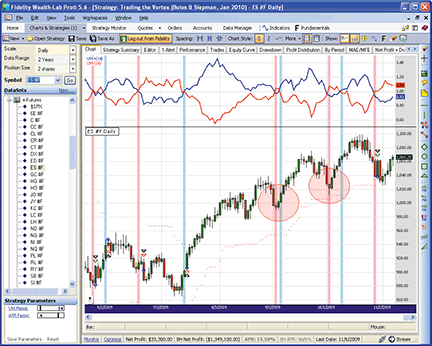Figure 3: WEALTH-LAB, VORTEX INDICATOR. The strategy to reverse on a stop was effective in remaining in strong trends despite multiple crossings of the Vortex Indicator.

```WealthScript Code (C#):

using System;
using System.Collections.Generic;
using System.Text;
using System.Drawing;
using WealthLab;
using WealthLab.Indicators;
using TASCIndicators;

namespace WealthLab.Strategies
{
public class MyStrategy : WealthScript
{
//Pushed indicator StrategyParameter statements
private StrategyParameter vmPeriod;
private StrategyParameter atrFactor;

public MyStrategy()
{
vmPeriod = CreateParameter("VM Period",14,5,100,10);
atrFactor = CreateParameter("ATR Factor",4,1,5,0.5);

}
protected override void Execute()
{
int period = vmPeriod.ValueInt;
int xBar = 0;      // Crossing bar or stop bar
bool bull = false;
double triggerPrice = -1d;
double stopPrice = -1d;

DataSeries vmPlus = VMPlus.Series(Bars ,period);
DataSeries vmMinus = VMMinus.Series(Bars ,period);
DataSeries atr = atrFactor.Value * ATR.Series(Bars, 10);
ChartPane paneVM = CreatePane(40,true,true);
PlotSeries(paneVM, vmPlus, Color.Blue, LineStyle.Solid, 2);
PlotSeries(paneVM, vmMinus, Color.Red, LineStyle.Solid, 2);
PlotStops();
HideVolume();

for(int bar = period; bar < Bars.Count; bar++)
{
if (CrossOver(bar, vmPlus, vmMinus))
{
SetBackgroundColor(bar, Color.LightBlue);
bull = true;
xBar = bar;
}
else if (CrossUnder(bar, vmPlus, vmMinus))
{
SetBackgroundColor(bar, Color.LightPink);
bull = false;
xBar = bar;
}

if (IsLastPositionActive)
{
Position p = LastPosition;
if (p.PositionType == PositionType.Long)
{
if (!bull)
{
SellAtStop(bar + 1, p, Low[xBar]);
ShortAtStop(bar + 1, Low[xBar]);
}
else if (SellAtTrailingStop(bar + 1, p, Close[bar] - atr[bar], "T-Stop"))
xBar = bar + 1;
}
else
{
if (bull)
{
CoverAtStop(bar + 1, p, High[xBar]);
BuyAtStop(bar + 1, High[xBar]);
}
else if (CoverAtTrailingStop(bar + 1, p, Close[bar] + atr[bar], "T-Stop"))
xBar = bar + 1;
}
}
else if (xBar > period) // initial and re-entry
{
if (bull) BuyAtStop(bar + 1, High[xBar]);
else ShortAtStop(bar + 1, Low[xBar]);
}
}
}
}
}
```

— Robert Sucher
www.wealth-lab.com

BACK TO LISTAMIBROKER: VORTEX INDICATOR

In “The Vortex Indicator” in this issue, Etienne Botes and Douglas Siepman present a new indicator that builds on some of the concepts first developed by J. Welles Wilder based on directional market movement.

Coding the Vortex Indicator is straightforward in AmiBroker. A ready-to-use formula for the article is presented here. To use it, enter this formula in the Afl Editor, then press the Insert Indicator button. You can click on the chart with the right mouse button and choose “Parameters” from the context menu to modify the period used for the Vortex Indicator. A sample chart is shown in Figure 4.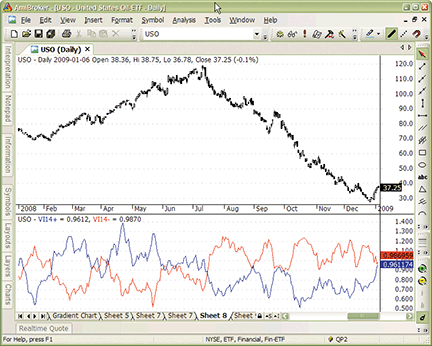Figure 4: AMIBROKER, VORTEX INDICATOR. Here is an example of a 14-day Vortex Indicator applied to USO (US Oil ETF).

```LISTING 1
// Vortex Indicator
// S&C Traders Tips Jan 2010
period = Param("Period", 14, 2 );

VMP = Sum( abs( H - Ref( L, -1 ) ), period );
VMM = Sum( abs( L - Ref( H, -1 ) ), period );
STR = Sum( ATR( 1 ), period );

VIP = VMP / STR;
VIM = VMM / STR;

Plot( VIP, "VI"+period+"+", colorBlue);
Plot( VIM, "VI"+period+"-", colorRed );
```

—Tomasz Janeczko, AmiBroker.com
www.amibroker.com

BACK TO LISTWORDEN BROTHERS STOCKFINDER: VORTEX INDICATOR

The Vortex Indicator described in Etienne Botes and Douglas Siepman’s article in this issue, “The Vortex Indicator,” has now been added to the library of indicators available in StockFinder.

You can add the indicator to your chart in StockFinder (Figure 5) by clicking the “Add indicator” button or by simply typing “/Vortex Indicator” and choosing it from the filtered list of available indicators.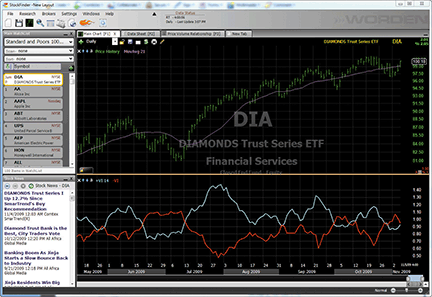Figure 5: STOCKFINDER, VORTEX INDICATOR

We have written the Vortex Indicator code in RealCode, which is based on the Microsoft Visual Basic.Net framework and uses the Visual Basic (VB) language syntax. RealCode is compiled into a .Net assembly and run by the StockFinder application. Unlike the scripting language that other trading applications use, RealCode is fully compiled and runs at the same machine-language level as the application itself. This gives you unmatched performance, with the flexibility of “run-time development and assembly” that you get by writing your own custom code.

Here is the code for the Vortex Indicator:

```'# Period = UserInput.Integer = 14
Static pVM As Single
Static nVM As Single
Static ATR As Single
If CurrentIndex > Period Then
pVM += (System.Math.Abs(Price.High - Price.Low(1)) - System.Math.Abs(Price.High(Period)
- Price.Low(Period + 1))) / Period
nVM += (System.Math.Abs(Price.Low - Price.High(1)) - System.Math.Abs(Price.Low(Period)
- Price.High(Period + 1))) / Period
ATR += (System.Math.Max(Price.High, Price.Last(1)) - System.Math.Min(Price.Low, Price.Last(1))
- System.Math.Max(Price.High(Period), Price.Last(Period + 1))
+ System.Math.Min(Price.Low(Period), Price.Last(Period + 1))) / Period
Else If isFirstBar Then
pVM = 0
nVM = 0
ATR = 0
Else
pVM += System.Math.Abs(Price.High - Price.Low(1)) / Period
nVM += System.Math.Abs(Price.Low - Price.High(1)) / Period
ATR += (System.Math.Max(Price.High, Price.Last(1)) - System.Math.Min(Price.Low, Price.Last(1))) / Period
End If
If ATR > 0 Then
Dim pVMnorm As Single = pVM / ATR
Dim nVMnorm As Single = nVM / ATR
OpenValue = nVMnorm
HighValue = System.Math.Max(pVMnorm, nVMnorm)
LowValue = System.Math.Min(pVMnorm, nVMnorm)
Plot = pVMnorm
Else
Plot = Single.NaN
End If
```

To download the StockFinder software and get a free trial, go to www.StockFinder.com.

— Bruce Loebrich and Patrick Argo
Worden Brothers, Inc.
www.StockFinder.com

BACK TO LISTOMNITRADER: VORTEX INDICATOR

For this month’s Traders’ Tip, we have coded the Vortex Indicator (VI) as described by Etienne Botes and Douglas Siepman in their article in this issue, “The Vortex Indicator.”

This indicator addresses some of the shortcomings found in the traditional average directional movement (Adx) calculation; in particular, it provides a significantly better treatment of inside bars.

In Figure 6, the Vortex Indicator is plotted on a chart of Aapl. Good long entries can be found when the VI+ line crosses above the VI- line. Similarly, short entries are signaled when VI- crosses above VI+.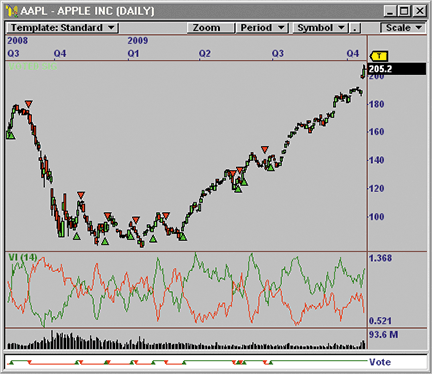Figure 6: OMNITRADER, VORTEX INDICATOR. Here is a demonstration of the Vortex Indicator (VI) and the signals generated by VI crossovers on a chart of Apple Inc. (AAPL).

To use this indicator, first copy the file “VI.txt” to the directory C:\Program Files\Nirvana\OT2010\VBA\Indicators. Next, open OmniTrader and click Edit:OmniLanguage. You should see “VI” in the Project Pane. Now simply click “compile,” and the code is ready to use. For more information and complete source code, visit www.omnitrader.com/ProSI.

```#Indicator
'**************************************************************
'*  Vortex Indicator (VI.txt)
'*   by Jeremy Williams and Matt Greenslet
'*	 November 11, 2009
'*
'*	Adapted from Technical Analysis of Stocks & Commodities
'*     January 2010
'*
'*  Summary:
'*
'*  This indicator addresses some of the shortcomings of the
'*  "The Vortex Indicator" in the January 2010 issue of Technical
'*  Analysis of Stocks & Commodities.
'*
'*  Parameters:
'*
'*  Periods -  Specifies the number of periods used for the calculation.
'*
'**************************************************************

#PARAM "Periods", 14, 1, 100

Dim ATRSum 		As Single
Dim VIPlus 		As Single
Dim VIMinus 	As Single

If Bar > Periods
'Calculate the Values of interest
ATRSum = Sum(ATR(1), Periods)
VIPlus = Sum(Abs(H - L), Periods)/ATRSum
VIMinus = Sum(Abs(L - H), Periods)/ATRSum

'Plot the VI+ and VI- lines
Plot("Vortex Plus", VIPlus)
Plot("Vortex Minus", VIMinus)
End If

' Return the difference between VI+ and VI-
Return VIPlus - VIMinus
```

—Jeremy Williams, Trading Systems Researcher
Nirvana Systems, Inc.

BACK TO LISTNEUROSHELL TRADER: VORTEX INDICATOR

The Vortex Indicator described by Etienne Botes and Douglas Siepman in their article in this issue can be easily implemented in NeuroShell Trader by combining a few of NeuroShell Trader’s 800+ indicators. First, select “New Indicator” from the Insert menu and use the Indicator Wizard to create the following indicators:

```+VM:	Abs( Subtract( High, Lag( Low, 1 ) ) )

-VM:	Abs( Subtract( Low, Lag( High, 1 ) ) )

TR:	Max3( Subtract( High, Low ),
Abs( Subtract( High, Lag( Close, 1) ) ),
Abs( Subtract( Low, Lag( Close, 1 ) ) ) )

VI+:	Divide( Sum( +VM, 14 ), Sum( TR, 14 ) )

VI-:	Divide( Sum( -VM, 14 ), Sum( TR, 14 ) )
```

Then, to create a trading system based on the Vortex Indicator, select “New Trading Strategy” from the Insert menu and enter the following in the appropriate locations of the Trading Strategy Wizard:

```Generate a buy long STOP when all of the following conditions are true:

A>B ( VI+, VI- )

Stop price:

SelectiveMovAvg ( High, CrossAbove( VI+, VI-), 1 )

Generate a short sell STOP when all of the following conditions are true:

A<B ( VI+, VI- )

Stop price:

SelectiveMovAvg ( Low, CrossBelow( VI+, VI-), 1 )```

If you have NeuroShell Trader Professional, you can also choose whether the system parameters should be optimized. After backtesting the trading strategy, use the “Detailed Analysis” button to view the backtest and trade-by-trade statistics for the system.

A sample chart is shown in Figure 7.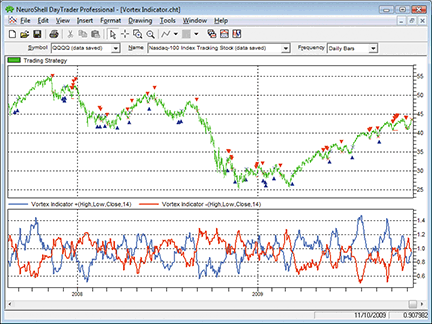Figure 7: NEUROSHELL TRADER, VORTEX INDICATOR. Here is a demonstration of a system based on the Vortex Indicator on the QQQQ.

—Marge Sherald, Ward Systems Group, Inc.
301 662-7950, sales@wardsystems.com
www.neuroshell.com

BACK TO LISTAIQ: VORTEX INDICATOR

The Aiq code is given here for the Vortex Indicator (VI) based on the article by Etienne Botes and Douglas Siepman in this issue, “The Vortex Indicator.”

The coded version I have supplied includes a system that can be used to test the indicator. The system is based only on the VI (or Dmi). To test the indicator in comparison to J. Welles Wilder’s directional movement indicator (Dmi), I devised a simple system based on crossovers of the VI (or Dmi). The rules for this long-only system are to go long when the VI (or Dmi) increases from below zero to above zero. The long positions are then exited when the VI (or Dmi) decreases from above zero to below zero.

I used the Portfolio Manager module to simulate trading a portfolio of stocks from the Nasdaq 100. I set the capitalization rules to invest 10% into each stock, taking no more than three new trades per day with up to 10 open positions at one time. When there were more than three signals per day, the ones with the highest VIplus (or DMIplus) values were chosen. All trades were simulated as placed “next day market on open.”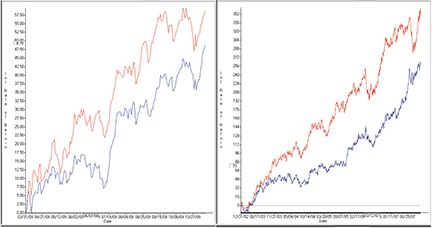Figure 8: AIQ SYSTEMS, VORTEX INDICATOR, BULL MARKET PERFORMANCE. Here, the equity curve for a VI system (blue line) is compared to the equity curve for a system based on J. Welles Wilder’s original DMI (red line) during two bull market periods, trading long only, using the NASDAQ 100 list of stocks.

In Figure 8, I show a comparison of two bull market periods, March 2009 through November 2009 and January 2002 through October 2007. The red line is the equity curve from a system based on J. Welles Wilder’s Dmi, while the blue line is the system based on the Vortex Indicator. Clearly, the original Dmi was the better performer during these two bull market periods. In Figure 9, I show a comparison of two bear market periods, October 2007 to March 2009 and March 2000 to December 2002. Again, the red line is the equity curve from the original Wilder Dmi system while the blue line is the VI system. In these two bear periods, the Vortex Indicator system shows a slight outperformance compared to the original Wilder Dmi. Based on this test, I would continue to use the original Dmi.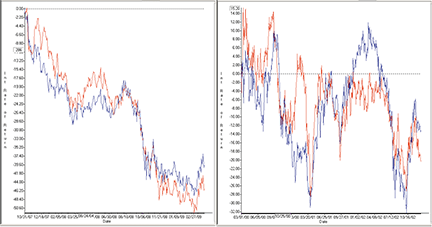Figure 9: AIQ SYSTEMS, VORTEX INDICATOR, BEAR MARKET PERFORMANCE. Here, the equity curve for a VI system (blue line) is compared to the equity curve for a system based on J. Welles Wilder’s original DMI (red line) during two bear market periods, trading long only, using the NASDAQ 100 list of stocks.

This code can be downloaded from the Aiq website at www.aiqsystems.com and also from www.TradersEdgeSystems.com/traderstips.htm.

```!  THE VORTEX INDICATOR
! Authors: Etienne Botes & Douglas Siepman, TASC January 2010
! Coded by: Richard Denning 11/06/09

vorLen is 14.
C is [close].
C1 is valresult(C,1).
H is [high].
H1 is valresult(H,1).
L is [low].
L1 is valresult(L,1).

sumVp is sum(abs(H - L1), vorLen).
sumVm is sum(abs(H1 - L),vorLen).
TR is Max(H - L,max(abs(C1 - L),abs(C1- H))).
sumTR is sum(TR, vorLen).
Vortex_Plus is sumVp / sumTR.    ! Plot with Vortex_Minus as
Vortex_Minus is sumVm / sumTR.   ! two line indicator
Vortex_Ind is Vortex_Plus-Vortex_Minus.! Plot as historigram

! SYSTEM TO TEST VORTEX COMPARED TO DMI

! DMI System:
BuyDMI if [dirmov] > 0 and valrule([dirmov] < 0,1).
SellDMI if [dirmov] < 0 and valrule([dirmov] > 0,1).
DMIplus is [+DMI].
DMIminus is [-DMI].

! VORTEX System:
BuyVI if Vortex_Ind > 0 and valrule(Vortex_Ind < 0,1).
SellVI if Vortex_Ind < 0 and valrule(Vortex_Ind > 0,1).
```

—Richard Denning
for AIQ Systems

BACK TO LISTTRADERSSTUDIO: VORTEX INDICATOR

The TradersStudio code is given here for the Vortex Indicator (VI), function, and system from the article in this issue, “The Vortex Indicator,” by Etienne Botes and Douglas Siepman.

The coded version that I have supplied also includes a system that can be used to test the indicator against J. Wells Wilder’s original directional movement index (Dmi). The system uses only the VI (or Dmi) and is based on crossovers of the VI (or Dmi). The rules for the system are:

1. Go long when the VI (or Dmi) goes from less than zero to greater than zero.
2. Go short when the VI (or Dmi) goes from above zero to less than zero.
3. All trades are placed “next day market on open.”

The system is always in the market either long or short. To test the indicator, I created a portfolio of 38 of the more actively traded, full-sized futures contracts. I used back-adjusted data (day session only) from Pinnacle Data for the following symbols: AD, BO, BP, C, CC, CD, CL, CT, DJ, DX, ED, FA, FC, FX, GC, HG, HO, HU, JO, JY, KC, KW, LC, LH, NG, NK, PB, RB, S, SB, SF, SI, SM, SP, TA, TD, UA, W.

A comparative test of the Vortex Indicator versus the original Dmi is shown on a year-by-year basis in the table in Figure 10. The test runs from 1978 to November 6, 2009. The years and metrics where the VI outperformed the Dmi are highlighted in light green. Over the entire test period and within the last 10 years, the VI shows a better performance than Wilder’s original Dmi when tested on this portfolio of futures markets using the 14-day parameter.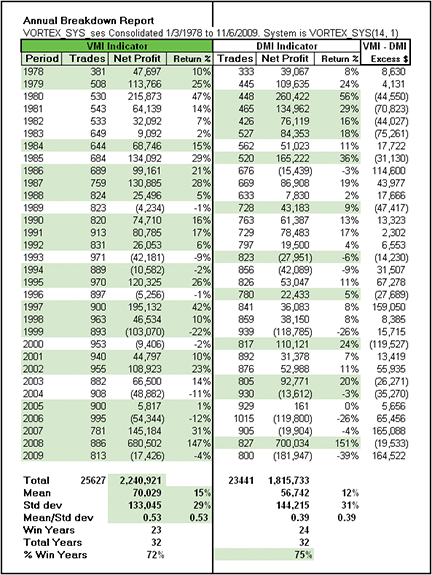Figure 10: TRADERSSTUDIO, VORTEX INDICATOR (VI) VS. DIRECTIONAL MOVEMENT INDEX (DMI), FUTURES, 1978—2009. This table shows a year-by-year comparison of the VI versus the DMI on a portfolio of 38 futures contracts trading one contract per trade. Light-green shaded areas highlight which indicator had the better performance.

I also ran a comparative test using 101 Nasdaq stocks over the period 1992 to 8/14/2009. These stocks were chosen based on high liquidity and high volatility and have similar characteristics to the stocks in the Nasdaq 100 index. Using this list of Nasdaq stocks showed the opposite results from the futures test in that the Dmi showed more profit and a higher mean to standard deviation ratio than the VI. This test on stocks is shown in Figure 11. These contradictory results indicate that further tests should be run.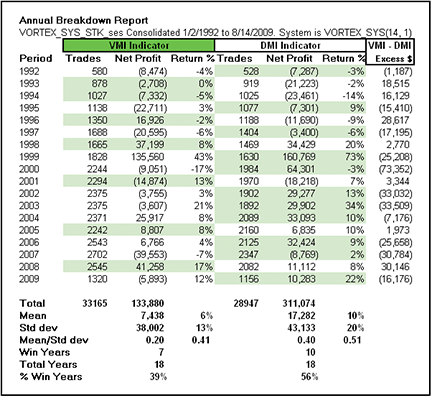Figure 11: TRADERSSTUDIO, VORTEX INDICATOR (VI) VS. DIRECTIONAL MOVEMENT INDEX (DMI), STOCKS, 1978—2009. This table shows a year-by-year comparison of the VI versus the DMI on a portfolio of 101 high-liquidity NASDAQ stocks trading 100 shares per trade. Light-green shaded areas highlight which indicator had the better performance.

```' THE VORTEX INDICATOR SYSTEM
' Authors: Etienne Botes & Douglas Siepman, TASC January 2010
' Coded by: Richard Denning 11/06/09

Sub VORTEX_SYS(vLen, useVortex)
' defaults: vLen = 14, useVortex = 1 (if set to <> 1 system
'   uses the original Wells Wilder DMI indicator)
Dim VI As BarArray
Dim VIp
Dim VIm
Dim DMI As BarArray

If useVortex = 1 Then
VI = VORTEX_INDEX(VIp,VIm,vLen)
If VI > 0 And VI < 0 Then Buy("LE_VORTEX",1,0,Market,Day)
If VI < 0 And VI > 0 Then Sell("SE_VORTEX",1,0,Market,Day)
Else
DMI = DMIplus(vLen,0) - DMIminus(vLen,0)
If DMI > 0 And DMI < 0 Then Buy("LE_DMI",1,0,Market,Day)
If DMI < 0 And DMI > 0 Then Sell("SE_DMI",1,0,Market,Day)
End If

End Sub
'---------------------------------------------------------------------
' THE VORTEX INDICATOR MULTI-OUTPUT FUNCTION
Function VORTEX_INDEX(ByRef VIp, ByRef VIm, vorLen)
Dim sumVMplus
Dim sumVMminus
Dim sumTR
Dim VIplus
Dim VIminus

sumVMplus = summ(Abs(H - L),vorLen)
sumVMminus = summ(Abs(H - L),vorLen)
sumTR = summ(TrueRange,vorLen)
If sumTR <> 0 Then
VIplus = sumVMplus / sumTR
VIminus = sumVMminus / sumTR
End If
VIp = VIplus
VIm = VIminus
VORTEX_INDEX = VIp - VIm
End Function
'---------------------------------------------------------------------
'THE VORTEX PLUS INDICATOR FUNCTION
Function VORTEX_PLUS(vorLen)
Dim sumVMplus As BarArray
Dim sumTR As BarArray

sumVMplus = summ(Abs(H - L),vorLen)
sumTR = summ(TrueRange,vorLen)
If sumTR <> 0 Then
VORTEX_PLUS = sumVMplus / sumTR
End If
End Function
'---------------------------------------------------------------------
'THE VORTEX MINUS INDICATOR FUNCTION
Function VORTEX_MINUS(vorLen)
Dim sumVMminus As BarArray
Dim sumTR As BarArray

sumVMminus = summ(Abs(H - L),vorLen)
sumTR = summ(TrueRange,vorLen)
If sumTR <> 0 Then
VORTEX_MINUS = sumVMminus / sumTR
End If
End Function
'---------------------------------------------------------------------
'THE VORTEX INDICATOR (FOR CHART DISPLAY)
sub VORTEX_IND(viLen)
plot1(VORTEX_PLUS(viLen))
plot2(VORTEX_MINUS(viLen))
End Sub
'---------------------------------------------------------------------
```

—Richard Denning

BACK TO LISTSTRATASEARCH: VORTEX INDICATOR

In their article in this issue, “The Vortex Indicator,” authors Etienne Botes and Douglas Siepman have given us a helpful revision to the directional movement index. And while our tests show that using this indicator had some significant limitations when used on its own, we found that the use of supporting indicators greatly improves its performance.

In our initial tests with the Vortex Indicator on its own, we used daily bars on the Nasdaq 100 component stocks. The system was profitable, but most of this profit was erased after adding a \$10 commission and a spread of 0.04. As the authors suggested, the system can create a fair number of false signals, and this was likely what we were seeing.

In our next test, we ran roughly 25,000 indicator combinations, with the Vortex Indicator being used alongside a large variety of supporting indicators. This created some impressive results, with annual returns exceeding 35%, percentage of profitable trades exceeding 65%, and drawdowns often staying below 20%. Clearly, this indicator can be a part of a winning system, but it may take the proper combination of supporting indicators to expose its true value.

As with all other Traders’ Tips, additional information, including plug-ins, can be found in the Shared Area of the StrataSearch user forum. This month’s plug-in allows StrataSearch users to run an automated search on the Vortex Indicator to help find effective supporting indicators to use.

A sample chart is shown in Figure 12.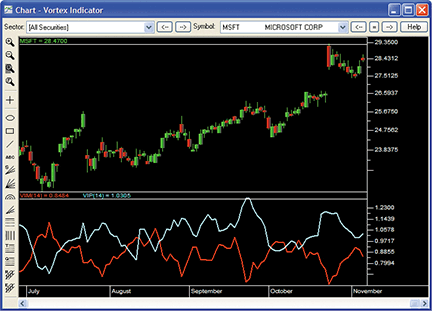Figure 12: STRATASEARCH, The Vortex Indicator. One method of using the Vortex Indicator is to buy when the +VI (blue line) crosses above the VI (red line) and sell when the +VI drops below the -VI.

```//*********************************************************
// Vortex Index Positive
//*********************************************************
days = parameter("Days");
E3 = abs(HIGH - ref(LOW, -1));
G16 = sum(E3, days);
I3 = higher(HIGH-LOW, higher(abs(HIGH-ref(CLOSE, -1)),
abs(LOW-ref(CLOSE, -1))));
J16 = sum(I3, days);
VIP = G16 / J16;

//*********************************************************
// Vortex Index Negative
//*********************************************************
days = parameter("Days");
F3 = abs(LOW - ref(HIGH, -1));
H16 = sum(F3, days);
I3 = higher(HIGH-LOW, higher(abs(HIGH-ref(CLOSE, -1)),
abs(LOW-ref(CLOSE, -1))));
J16 = sum(I3, days);
VIM = H16 / J16;
```

—Pete Rast
Avarin Systems, Inc.
www.StrataSearch.com

BACK TO LISTTRADECISION: VORTEX INDICATOR

The article by Etienne Botes and Douglas Siepman in this issue, “The Vortex Indicator,” demonstrates a technical indicator for trading a change in market direction. The Vortex Indicator was developed as a new type of directional movement indicator by drawing inspiration from J. Welles Wilder’s original directional movement index (Dmi) as well as from Viktor Schauberger’s study of the vortex motions of water.

To implement the VI in Tradecision, use the Indicator Builder to set up two indicators: Vortex Indicator Plus and Vortex Indicator Minus. Here is the Tradecision code for the Vortex Indicator Plus:

```Vortex Indicator Plus:
input
Length:"Length",14;
end_input

var
VMPlus:=0;
VMPlusS:=0;
VMTR:=0;
VMTRS:=0;
VIPlus:=0;
end_var

VMPlus:= Abs(High - Low\1\);
VMPlusS:=CumSum(VMPlus,Length);

VMTR:= Max(VMPlus, Abs(High - Close\1\), Abs(Low - Close\1\));  VMTRS:=CumSum(VMTR,Length);

VIPlus:= VMPlusS/VMTRS;

return VIPlus;
```

Here is the Tradecision code for the Vortex Indicator Minus:

```Vortex Indicator Minus:
input
Length:"Length",14;
end_input

var
VMPlus:=0;
VMMinus:=0;
VMMinusS:=0;
VMTR:=0;
VMTRS:=0;
VIMinus:=0;
end_var

VMPlus:= Abs(High - Low\1\);
VMMinus:=Abs(Low - High\1\);
VMMinusS:=CumSum(VMMinus,Length);

VMTR:= Max(VMPlus, Abs(High - Close\1\), Abs(Low - Close\1\));  VMTRS:=CumSum(VMTR,Length);

VIMinus:= VMMinusS/VMTRS;

return VIMinus;
```

To import the strategy into Tradecision, visit the area “Traders' Tips from Tasc Magazine” at www.tradecision.com/support/tasc_tips/tasc_traders_tips.htm or copy the code from the Stocks & Commodities website at www.traders.com. A sample chart implementation is shown in Figure 13.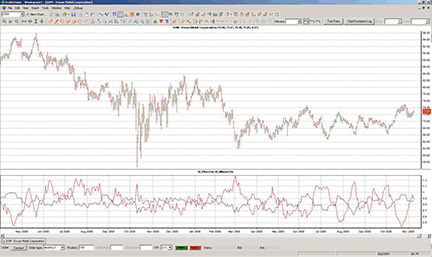FIGURE 13: TRADECISION, 14-PERIOD DAILY VORTEX INDICATOR PLOTTED ON AN XOM DAILY CHART. +VI and -VI converge and diverge in relation to one another and sometimes intersect each other. The crossing points are the potential trend change points.

—Yana Timofeeva, Alyuda Research

BACK TO LISTTRADINGSOLUTIONS: VORTEX INDICATOR

In “The Vortex Indicator” in this issue, Etienne Botes and Douglas Siepman combine concepts from J. Welles Wilder’s directional movement index (Dmi) with concepts from flow dynamics to create a pair of indicators that highlight positive and negative trend movement.

The TradingSolutions functions based on their article are given here and are also available as a function file that can be downloaded from the TradingSolutions website (www.tradingsolutions.com) in the “free systems” section.

```Function Name: Vortex Indicator Plus (VI+)
Short Name: VIPlus
Inputs: Close, High, Low, Period
Div (Sum (Abs (Sub (High, Lag (Low, 1))), Period), Sum (TR (Close, High, Low), Period))

Function Name: Vortex Indicator Minus (VI-)
Short Name: VIMinus
Inputs: Close, High, Low, Period
Div (Sum (Abs (Sub (Low, Lag (High, 1))), Period), Sum (TR (Close, High, Low), Period))
```

—Gary Geniesse
NeuroDimension, Inc.
800 634-3327, 352 377-5144

BACK TO LISTNEOTICKER: VORTEX INDICATOR

The Vortex Indicator as presented by Etienne Botes and Douglas Siepman in their article in this issue can be implemented in NeoTicker as a formula language indicator (Listing 1) with period as a parameter. This parameter will allow users to easily adjust the period on which the Vortex Indicator is calculated. The Vortex Indicator will return VI+ and VI- calculations and plot the values in separate panes on the chart (Figure 14).

Within the indicator code, I substituted a direct true range calculation as shown in the article’s Excel sidebar with the built-in truerange. This will improve execution speed and efficiency of the indicator. SafeDiv is a formula function that avoids a division-by-zero error.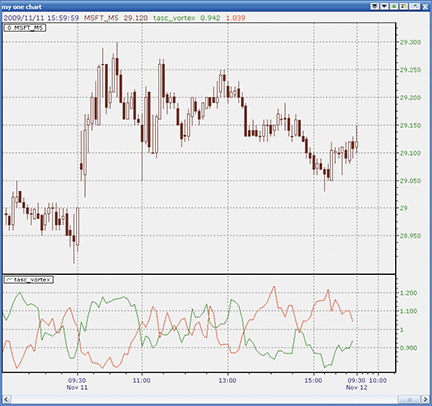Figure 14: NEOTICKER, VORTEX INDICATOR. The NeoTicker indicator code will return a VI+ and VI- calculation and plot the values in separate panes on the chart.

A downloadable version of the indicator and a sample system implementation using the Vortex Indicator will be available in the NeoTicker blog (http://blog.neoticker.com).

```LISTING 1

VMPlus  := absvalue(high-low(1));
VMMinus := absvalue(low-high(1));
\$TR_sum := summation(truerange(data1), param1);
\$VMPlus_sum := summation(VMPlus, param1);
\$VMMinus_sum := summation(VMMinus, param1);
plot1 := SafeDiv(\$VMPlus_sum,  \$TR_sum, 0);
plot2 := SafeDiv(\$VMMinus_sum, \$TR_sum, 0);
```

—Kenneth Yuen
TickQuest, Inc.
www.neoticker.com

BACK TO LISTUPDATA: VORTEX INDICATOR

This tip is based on Etienne Botes and Douglas Siepman’s article in this issue, “The Vortex Indicator.”

Taking principles from J. Welles Wilder’s directional movement index (Dmi), the authors seek to recreate the vortexes displayed in fluid dynamics to determine data trends across varied time frames. The Updata team has found this indicator, in combination with the simple entry/exit conditions included in our code, to work particularly well across European stock indexes.

The Updata code for this system has been entered in the Updata System Library and may be downloaded by clicking the Custom menu and then System Library. Those who cannot access the library due to firewall issues may paste the following code into the Updata custom editor and save it.

```NAME VORTEX INDICATOR
PARAMETER "PERIOD" #PERIOD=14
DISPLAYSTYLE 2LINES
PLOTSTYLE LINE RGB(0,0,255)
PLOTSTYLE2 LINE RGB(255,0,0)
INDICATORTYPE CHART NEWWINDOW
INDICATORTYPE2 CHART SUPERIMPOSERIGHT

@pVM=0
@nVM=0
#I=0
@SUMpVM=0
@SUMnVM=0
@SUMTR=0
@ATR=0
@pVI=0
@nVI=0
@LONG=FALSE
@LONGENTRY=0
@SHORT=FALSE
@SHORTENTRY=0
@LONGSTOP=0
@SHORTSTOP=0

FOR #CURDATE=0 TO #LASTDATE

@SUMpVM=0
@SUMnVM=0
@SUMTR=0
@pVM=ABS(HIGH-LOW(1))
@nVM=ABS(LOW-HIGH(1))
@ATR=ATR(1)

IF #CURDATE>#PERIOD

'ENTRIES
IF @LONG=TRUE AND OPEN>@LONGENTRY
@LONG=FALSE
ELSEIF @LONG=TRUE AND HIGH>@LONGENTRY
@LONG=FALSE
ELSEIF @SHORT=TRUE AND OPEN<@SHORTENTRY
SHORT OPEN
@SHORT=FALSE
ELSEIF @SHORT=TRUE AND LOW<@SHORTENTRY
SHORT @SHORTENTRY
@SHORT=FALSE
ENDIF

FOR #I=0 TO (#PERIOD-1)
@SUMpVM=@SUMpVM+HIST(@pVM,#I)
@SUMnVM=@SUMnVM+HIST(@nVM,#I)
@SUMTR=@SUMTR+HIST(@ATR,#I)
NEXT

'VORTEX INDICATOR
@pVI=@SUMpVM/@SUMTR
@nVI=@SUMnVM/@SUMTR

'ENTRY SETUP
IF HASX(@pVI,@nVI,UP) AND @LONG=FALSE
@LONG=TRUE
@SHORT=FALSE
@LONGENTRY=HIGH
ELSEIF HASX(@pVI,@nVI,DOWN) AND @SHORT=FALSE
@SHORT=TRUE
@LONG=FALSE
@SHORTENTRY=LOW
ENDIF

'MARKET DERIVED EXITS: TRAILING STOPS AND PROFIT TARGETS
IF ORDERISOPEN=1 AND LOW<LOW(1)
SELL LOW(1)
ELSEIF ORDERISOPEN=-1 AND HIGH>HIGH(1)
COVER HIGH(1)
ENDIF

@PLOT=@pVI
@PLOT2=@nVI

ENDIF

NEXT

```

A sample chart is shown in Figure 15.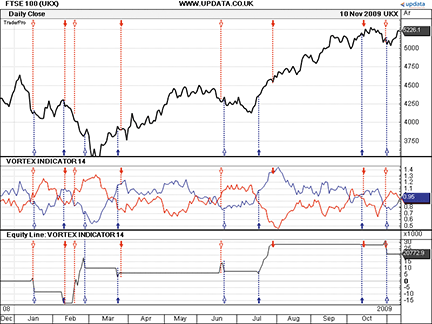FIGURE 15: UPDATA, VORTEX INDICATOR. This chart shows the Vortex Indicator on the FTSE 100 Index.

—Updata Support team
Support@updata.co.uk
www.updata.co.uk

BACK TO LISTWAVE59: VORTEX INDICATOR

In their article in this issue, “The Vortex Indicator,” Etienne Botes and Douglas Siepman present their Vortex Indicator, an improved version of J. Welles Wilder’s classic indicator, the directional movement index (Dmi).

Figure 16 is a weekly chart of Qqqq showing the Vortex Indicator in action. Note the timely call for a low in March 2009 as well as the very bullish indication since that time. More important, note that the VM+ is preparing to cross under the VM-, which would indicate a change in trend to the downside.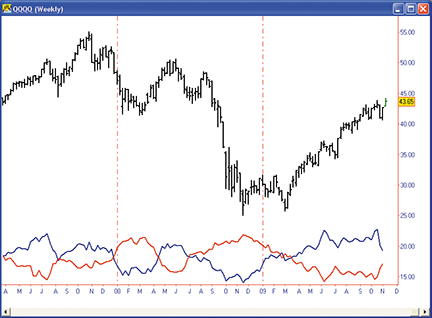FIGURE 16: WAVE59, VORTEX INDICATOR. Shown is a weekly chart of QQQQ with the Vortex Indicator. Note the timely call for a low in March 2009 as well as the very bullish indication since then. More important, note that the VM+ is preparing to cross under the VM-, which would indicate a change in trend to the downside.

The following script implements this indicator in Wave59. As always, users of Wave59 can download these scripts directly using the QScript Library found at http://www.wave59.com/library.

```Indicator:  SC_BotesSiepman_Vortex
input:length(14),plus_color(blue),minus_color(red),thickness(1);

#calculate raw vm+ and vm-
vm_plus = abs(high-low);
vm_minus = abs(low-high);

#sum vm+ and vm-
sum_vm_plus = summation(vm_plus,length);
sum_vm_minus = summation(vm_minus,length);

#calculate true range
sum_tr = summation(truerange(),length);

#divide summed vm+, vm- by summed true range
vm_plus_final = sum_vm_plus/sum_tr;
vm_minus_final = sum_vm_minus/sum_tr;

#plot it
plot1 = vm_plus_final;
plot2 = vm_minus_final;
color1 = plus_color;
color2 = minus_color;
thickness1, thickness2 = thickness;
```

—Earik Beann
Wave59 Technologies Int’l, Inc.
www.wave59.com

BACK TO LISTTRADE NAVIGATOR: VORTEX INDICATOR

Trade Navigator offers everything needed for recreating the indicators discussed in “The Vortex Indicator” by Etienne Botes and Douglas Siepman in this issue. We will demonstrate how to create the necessary custom indicators and then add them to any chart in Trade Navigator.

Use the following TradeSense code to set up the custom indicators. First, open the Trader’s Toolbox, click on the Functions tab and click the New button.

`VIPlus`

Input the following code:

```&VMplus := Abs (High - Low.1)
&VMminus := Abs (Low - High.1)
&VMplus14 := MovingSum (&VMplus , 14 , 0)
&VMminus14 := MovingSum (&VMminus , 14 , 0)
&TR := True Range
&TR14 := MovingSum (&TR , 14 , 0)

&VI14plus := &VMplus14 / &TR14
&VI14minus := &VMminus14 / &TR14
&VI14plus
```

Click the Verify button. When you are finished, click on the Save button, type a name for your new function and click OK.

Repeat these steps for the VIMinus function using the following formula for each:

```&VMplus := Abs (High - Low.1)

&VMminus := Abs (Low - High.1)
&VMplus14 := MovingSum (&VMplus , 14 , 0)
&VMminus14 := MovingSum (&VMminus , 14 , 0)
&TR := True Range
&TR14 := MovingSum (&TR , 14 , 0)
&VI14plus := &VMplus14 / &TR14

&VI14minus := &VMminus14 / &TR14
&VI14minus
```

To display the indicators on a chart, go to the “Add to chart” window by clicking on the chart and typing “A” on the keyboard. Click on the Indicators tab, find the VIPlus indicator in the list and either doubleclick on it or highlight the name and click the “Add” button.

Repeat these steps to add VIMinus. Once you have the indicators added to the chart, click on the label for VIMinus and drag it into the pane containing VIPlus.

Highlight each indicator and change it to the desired color in the chart settings window. When you have them the way you want to see them, click OK.

You can save your new chart as a template to use for other charts. Once you have the chart set up, go to the Charts dropdown menu, select Templates and then “Manage chart templates.” Click on the New button, type in a name for your new template and click OK.

Genesis Financial Technologies has provided a library called “Stocks & Commodities Vortex” that includes a template with these custom indicators in a special file named “SC0110,” downloadable through Trade Navigator.

—Michael Herman
Genesis Financial Technologies
www.GenesisFT.com

BACK TO LISTINVESTOR/RT: VORTEX INDICATOR

The Vortex Indicator as described by Etienne Botes and Douglas Siepman in their article in this issue can be implemented in Investor/RT using two custom indicators. The syntax for the +VI custom indicator is simply:

`SUM(HI - LO.1, 14) / SUM(TR, 14)`

and the syntax for the -VI custom indicator is:

`SUM(HI.1 - LO, 14) / SUM(TR, 14)`

These calculations are based on a 14-period Vortex Indicator. To change the period, simply change the number “14” to the new period in the two locations it is found in each custom indicator. A chart showing the +VI and -VI on daily bars of the S&P mini contract can be seen in Figure 17.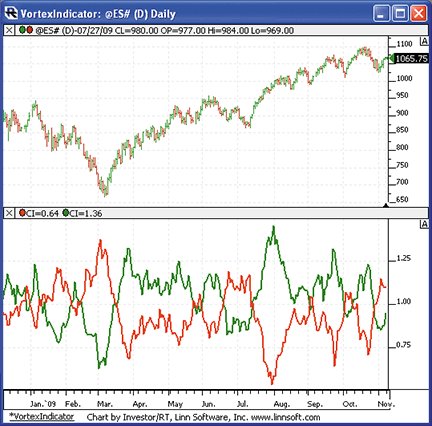FIGURE 17: INVESTOR/RT, Vortex Indicator. A 14-period Vortex Indicator is plotted on daily bars of the S&P mini contract. The green line in the lower pane represents the +VI while the red line represents the -VI.

To import a chart containing the Vortex Indicator into Investor/RT, visit http://www.linnsoft.com/charts/VortexIndicator.html

To learn more about creating custom indicators in Investor/RT, visit http://www.linnsoft.com/tutorials/rtl.htm.

Linn Software
info@linnsoft.com,
www.linnsoft.com

BACK TO LISTNINJATRADER: VORTEX INDICATOR

The Vortex Indicator as discussed in Etienne Botes and Douglas Siepman’s article in this issue has been implemented as an indicator available for download at www.ninjatrader.com/SC/January2010SC.zip.

Once it has been downloaded, from within the NinjaTrader Control Center window, select the menu File → Utilities → Import NinjaScript and select the downloaded file. This strategy is for NinjaTrader version 6.5 or greater.

You can review the indicator’s source code by selecting the menu Tools → Edit NinjaScript → Indicator from within the NinjaTrader Control Center window and selecting “VortexIndicator.”

NinjaScript indicators are compiled Dlls that run native, not interpreted, which provides you with the highest performance possible.

A sample chart implementing the strategy is shown in Figure 18.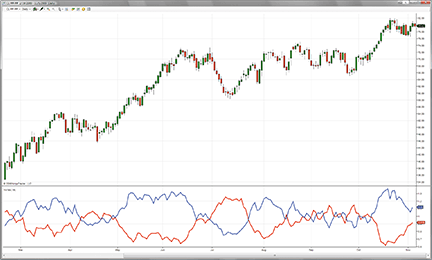Figure 18: NINJATRADER, VORTEX INDICATOR. This sample chart shows the Vortex Indicator applied to a daily chart of crude oil.

—Raymond Deux & Austin Pivarnik

BACK TO LISTVT TRADER: VORTEX INDICATOR

Our Traders’ Tip submission is inspired by the article “The Vortex Indicator” by Etienne Botes and Douglas Siepman in this issue. We’ll be offering the Vortex Indicator for download in our online forums.

The VT Trader code and instructions for recreating the indicator are as follows:

1. VT Trader’s Ribbon→Technical Analysis menu→Indicators group→Indicators Builder→[New] button
2. In the General tab, type the following text into each corresponding text box:
```Name: TASC - 01/2010 - Vortex Indicator
Function Name Alias: tasc_Vortex
Label Mask: TASC - 01/2010 - Vortex Indicator (%Periods%)
+VI = %PlusVI%, -VI = %MinusVI%
Placement: New Frame
Data Inspection Alias: Vortex
```
3. In the Input Variable(s) tab, create the following variables:
```[New] button...
Name: Periods
Display Name: Periods
Type: integer
Default: 13

```
4. In the Output Variable(s) tab, create the following variables:
```[New] button...
Var Name: PlusVI
Name: (Plus VI)
Line Color: blue
Line Width: slightly thicker
Line Type: solid

[New] button...
Var Name: MinusVI
Name: (Minus VI)
Line Color: red
Line Width: slightly thicker
Line Type: solid
```
5. In the Formula tab, copy and paste the following formula:
```{Provided By: Capital Market Services, LLC & Visual Trading Systems, LLC}
{Description: TASC, January 2010 - "Reliability is Harder than it Looks  - The Vortex Indicator" }
{by Etienne Botes and Douglas Siepman}
{File: tasc_Vortex.vtscr - Version 1.0}

PlusVM:= abs(H-ref(L,-1));
MinusVM:= abs(L-ref(H,-1));

SumPlusVM:= sum(PlusVM,Periods);
SumMinusVM:= sum(MinusVM,Periods);

TrueRange:= max(H-L,max(abs(H-ref(C,-1)),abs(L-ref(C,-1))));
SumTrueRange:= sum(TrueRange,Periods);

PlusVI:= SumPlusVM/SumTrueRange;
MinusVI:= SumMinusVM/SumTrueRange;
```
6. Click the “Save” icon in the toolbar to finish building the Vortex Indicator.

To attach the indicator to a chart (Figure 19), click the right mouse button within the chart window and then select “Add Indicator” → “Tasc - 01/2010 - Vortex Indicator” from the indicator list.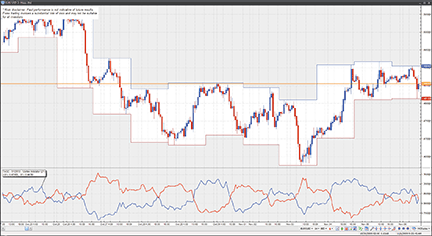Figure 19: VT TRADER, VORTEX INDICATOR. Here is the Vortex Indicator on a one-hour candlestick chart of the EUR/USD.

Risk disclaimer: Past performance is not indicative of future results. Forex trading involves a substantial risk of loss and may not be suitable for all investors.

—Chris Skidmore
CMS Forex
www.cmsfx.com

BACK TO LISTTRACK ‘N TRADE PRO: AVERAGE DRECTIONAL MOVEMENT INDEX (ADX)

Developed by J. Welles Wilder and explained in his book first published in 1978, New Concepts In Technical Trading Systems, the directional movement index (Dmi) can be used by itself or as a filter on a trend-following system. The Dmi helps determine whether a market is trending.

In a Dmi study, three lines are generated: DM+, DM-, and Adx. The DM+ line measures positive (upward) movement or buying pressure and the DM- number measures negative (downward) movement, reflecting selling pressure. The Adx measures the divergence between the DM+ and DM-.

Buy signals are generated when the DM+ crosses above the DM-, and sell signals are generated when the DM- crosses above the DM+.

Wilder also suggests that when a crossover occurs, the extreme price (that is, the high for a buy or low for a sell made during the trading interval of the crossover) can be interpreted as a breakout point. Wilder also recommended that DM+/DM- crossover signals only be taken when the Adx is above both the DM+ and DM-, or a specific threshold. Note that Track ‘n Trade Pro allows traders to set up to four different threshold levels for Adx as well as generate buy and sell signals using extreme points on crossovers.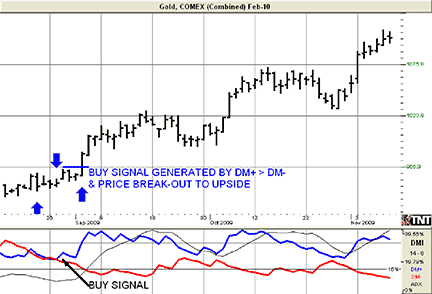FIGURE 20: TRACK ‘N TRADE, DIRECTIONAL MOVEMENT INDEX

Calculating DMI
To calculate Dmi, you must first compute the directional movement, DM, for the current trading interval. Directional movement can be up, down, or zero. If directional movement is up, it is labeled as +DM, while -DM refers to downward directional movement.

`Hight - Hight-1 or Lowt - Lowt-1`

The next step in determining the Dmi is to compute the true range. The true range (TR) is always a positive number. According to Wilder, the true range is the largest value of three equations:

```Hight - Lowt
Hight - Closet-1
Lowt - Closet-1
```

Using the above calculations, the formula can be simplified as follows:

```+DMt = (+DMt-1 - (+DMt-1 / n)) + (+DMt)
-DMt = (-DMt-1 - (-DMt-1 / n)) + (-DMt)
TRt = (TRt-1 - (TRt-1 / n)) + (TRt)
```

You now have the average values. The next step is to compute the directional indicator. It can be either up or down, depending on the directional movement. On up intervals, use this calculation:

`+DI = (+DM / TR) x 100 `

On a down interval, use this formula:

`-DI = (-DM / TR) x 100 `

You compute the difference between the +DI and the -DI. Remember to use the absolute value of this difference (that is, convert any negative value into a positive number).

`DIdiff = | ((+DI) - (-DI)) | `

Compute the sum of the directional indicator values using this formula:

`DIsum = ((+DI) + (-DI)) `

Once you compute the DIdiff and the DIsum, you can calculate the DX or directional movement index. This value is always a percentage:

`DX = (DIdiff / DIsum) x 100 `

The result is the Adx or average directional movement index. This is the computational procedure:

`ADXt = ( (ADXt-1 x (n - 1) ) + DXt) / n`

Adx by itself can be a useful confirmation indicator, as a low Adx reading is indicative of a weak trending market. Under such circumstances, traders should look to use overbought/oversold indicators, such as stochastics, Rsi, and so on. When Adx is rising or is above a specific threshold, traders should look to use trend-following indicators like Macd, momentum, and moving averages.

—Scott W. Barrie
For Gecko Software
www.geckosoftware.com

BACK TO LISTMETASTOCK: VORTEX INDICATOR

The article “The Vortex Indicator” introduces a new indicator and a suggested trading method. Both can be added to MetaStock with the formulas listed below.

I split the indicator into two separate formulas to make it easier to color them correctly. To enter these indicators into MetaStock:

1. In the Tools menu, select Indicator Builder.
2. Click New to open the Indicator Editor for a new indicator.
3. Type the name “Vortex +”.
4. Click in the larger window and paste or type in the formula:

```tp:= Input("time periods",1,100,14);
Sum(Abs(H-Ref(L,-1)),tp)/
Sum(Max(H,Ref(C,-1))-Min(L,Ref(C,-1)),tp)```

5. Click Ok to close the Indicator Editor.
6. Click New to open the Indicator Editor for a new indicator.
7. Type the name “Vortex -”.
8. Click in the larger window and paste or type in the formula:

```tp:= Input("time periods",1,100,14);
Sum(Abs(L-Ref(H,-1)),tp)/
Sum(Max(H,Ref(C,-1))-Min(L,Ref(C,-1)),tp)```

9. Click Ok to close the Indicator Editor.
10. Click Ok to close Indicator Builder.

The system test and instructions for creating it in MetaStock are:

1. Select Tools > the Enhanced System Tester.
2. Click New.
3. Enter a name, “Vortex System”.
4. Select the Buy Order tab and enter the following formula:

```tp:= 14;
vip:=Sum(Abs(H-Ref(L,-1)),tp)/
Sum(Max(H,Ref(C,-1))-Min(L,Ref(C,-1)),tp);
vim:=Sum(Abs(L-Ref(H,-1)),tp)/
Sum(Max(H,Ref(C,-1))-Min(L,Ref(C,-1)),tp);
bset:=cross(vip,vim);
cross(h,valuewhen(1,bset,h))```

5. Set the Order Type to Stop.
6. Select the Limit or Stop Price and enter the following formula:

```tp:= 14;
vip:=Sum(Abs(H-Ref(L,-1)),tp)/
Sum(Max(H,Ref(C,-1))-Min(L,Ref(C,-1)),tp);
vim:=Sum(Abs(L-Ref(H,-1)),tp)/
Sum(Max(H,Ref(C,-1))-Min(L,Ref(C,-1)),tp);
bset:=cross(vip,vim);
valuewhen(1,bset,h)```

7. Select the Sell Order tab and enter the following formula:

```tp:= 14;
vip:=Sum(Abs(H-Ref(L,-1)),tp)/
Sum(Max(H,Ref(C,-1))-Min(L,Ref(C,-1)),tp);
vim:=Sum(Abs(L-Ref(H,-1)),tp)/
Sum(Max(H,Ref(C,-1))-Min(L,Ref(C,-1)),tp);
sset:=cross(vim,vip);
cross(valuewhen(1,sset,L),L)```

8. Set the Order Type to Stop.
9. Select the Limit or Stop Price and enter the following formula:

```tp:= 14;
vip:=Sum(Abs(H-Ref(L,-1)),tp)/
Sum(Max(H,Ref(C,-1))-Min(L,Ref(C,-1)),tp);
vim:=Sum(Abs(L-Ref(H,-1)),tp)/
Sum(Max(H,Ref(C,-1))-Min(L,Ref(C,-1)),tp);
sset:=cross(vim,vip);
valuewhen(1,sset,L)```

10. Select the Sell Short Order tab and enter the following formula:

```tp:= 14;
vip:=Sum(Abs(H-Ref(L,-1)),tp)/
Sum(Max(H,Ref(C,-1))-Min(L,Ref(C,-1)),tp);
vim:=Sum(Abs(L-Ref(H,-1)),tp)/
Sum(Max(H,Ref(C,-1))-Min(L,Ref(C,-1)),tp);
sset:=cross(vim,vip);
cross(valuewhen(1,sset,L),L)```

11. Set the Order Type to Stop.
12. Select the Limit or Stop Price and enter the following formula:

```tp:= 14;
vip:=Sum(Abs(H-Ref(L,-1)),tp)/
Sum(Max(H,Ref(C,-1))-Min(L,Ref(C,-1)),tp);
vim:=Sum(Abs(L-Ref(H,-1)),tp)/
Sum(Max(H,Ref(C,-1))-Min(L,Ref(C,-1)),tp);
sset:=cross(vim,vip);
valuewhen(1,sset,L)```

13. Select the Buy to Cover Order tab and enter the following formula:

```tp:= 14;
vip:=Sum(Abs(H-Ref(L,-1)),tp)/
Sum(Max(H,Ref(C,-1))-Min(L,Ref(C,-1)),tp);
vim:=Sum(Abs(L-Ref(H,-1)),tp)/
Sum(Max(H,Ref(C,-1))-Min(L,Ref(C,-1)),tp);
bset:=cross(vip,vim);
cross(h,valuewhen(1,bset,h))```

14. Set the Order Type to Stop.
15. Select the Limit or Stop Price and enter the following formula:

```tp:= 14;
vip:=Sum(Abs(H-Ref(L,-1)),tp)/
Sum(Max(H,Ref(C,-1))-Min(L,Ref(C,-1)),tp);
vim:=Sum(Abs(L-Ref(H,-1)),tp)/
Sum(Max(H,Ref(C,-1))-Min(L,Ref(C,-1)),tp);
bset:=cross(vip,vim);
valuewhen(1,bset,h)```

16. Click OK to close the system editor.

—William Golson
Equis International
www.MetaStock.com

BACK TO LISTTHE VORTEX INDICATOR WITH EXCEL — ARTICLE CODE

Traders will recognize that the calculation for the Vortex Indicator is in many ways similar to J. Welles Wilder’s Dmi. In the Excel spreadsheet (Figure 21), the first calculation is for the positive trending vortex movement +VM column and starts in cell E3:

`= ABS(B3-C2)`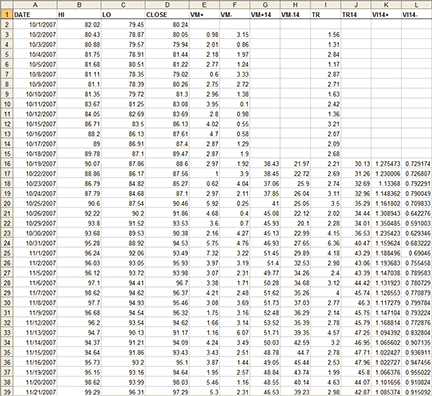FIGURE 21: CALCULATING THE VORTEX INDICATOR USING AN EXCEL SPREADSHEET

Column F calculates the negative trending vortex movement VM. The formula for cell F3 is:

`=ABS(C3-B2)`

The daily calculations are volatile so the data needs to be smoothed. This is done by deciding on a parameter length for the Vortex Indicator. For this example, we have chosen a 14-period vortex. The formula is simply the sum of the last 14 +VM values in cell G16:

`=SUM(E3:E16)`

The same for the VM values in cell H16:

`=SUM(F3:F16)`

Next, we calculate the true range (TR) in cell I3:

`=MAX(B3-C3,ABS(B3-D2),ABS(C3-D2))`

Next, we need to get the sum of the last 14 periods’ true range. Keep in mind if you want to change the parameter of vortex to 21, 34, or 55, you will also need to use the sum of the last 21, 34, or 55 periods’ true range. In this case, we sum the true range in cell J16:

`=SUM(I3:I16)`

Finally, we need to calculate the ratio of +VM14 and -VM14 to the sum of the last 14 days’ daily true ranges. We do this in cell K16:

`=G16/J16`

Similarly, for cell L16:

`=H16/J16`

At this point, these two last columns can be used to draw the graph of the Vortex Indicator.

—Etienne Botes & Douglas Siepman
etienne@vortexfund.com, douglas@vortexfund.com
www.vortexfund.com

BACK TO LIST